# Homology manifold

(diff) ← Older revision | Latest revision (diff) | Newer revision → (diff)

generalized manifold

A locally compact topological space whose local homological structure is analogous to the local structure of ordinary topological manifolds, including manifolds with boundary. More exactly, a homology-manifold (a generalized-manifold) over a group or a moduleof coefficients is a locally compact topological spacewith finite homological dimension (cf. Homological dimension of a space) overand such that all its local homology groups (cf. Local homology)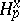are trivial if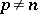, and are isomorphic either toor zero if. Here,is the direct limit of the groups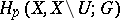, taken over all neighbourhoodsof the point, andis a homology theory that satisfies all the Steenrod–Eilenberg axioms, including the exactness axiom. In the category of locally contractible spaces the theory, considered with compact support, is isomorphic to the singular theory (cf. Singular homology). The groups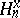automatically turn out to be the stalks of some sheaf(cf. Sheaf theory), known as the orienting sheaf of the manifold. A homology manifoldis said to be orientable if the sheaf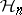is isomorphic to the constant sheaf, and is said to be locally orientable ifis locally constant at the points where. Ifis a principal ideal ring and if allare non-zero, a homology manifold overis always locally orientable. If a homology manifold over a groupis locally orientable, then the set of allon which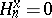is closed, nowhere dense and forms the boundary of the homology manifold. A locally orientable homology manifoldhas the same homological properties as ordinary manifolds.

E.g., the theorem on preservation of domain is valid for,, the set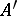is nowhere dense inif and only if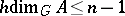, etc.

For any homology manifold overthere are natural isomorphisms (Poincaré duality)(cohomology with coefficients in a sheaf). Hereis any integer; however, the homological dimension of the homology manifoldoveris, and thus the content of these isomorphisms is non-trivial only if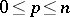. Similar isomorphisms are valid for homology and cohomology with support in any paracompactifying family (in particular, for homology and cohomology spaces with compact support). The condition of isomorphism between the non-zero stalksof the sheafand the groupis immaterial. Instead of the groupit is also possible to consider any locally constant sheaf of coefficients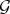with stalk(this is accompanied by a change in). Any open subset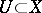is a homology manifold. For this reason, the use of the equations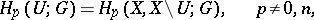in the second one of whichhas compact closure, while the indexindicates the compactness of the supports, makes it possible to obtain the isomorphisms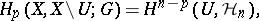as special cases of Poincaré duality. Combination of the exact homology and cohomology sequences of the respective pairs also makes it possible to consider the isomorphisms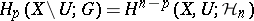and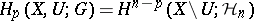— the latter one being a generalization of Alexander duality — as special cases of Poincaré duality. Similar relations are also valid for homology and cohomology with supports in a given fixed family.

Let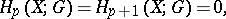letbe compact and letbe a closed or an open subset. A consequence of the previous isomorphisms and of the exactness of the homology and cohomology is an isomorphismwhich represents the Pontryagin duality for a closedand the Steenrod duality for an open. This and the property of continuity of cohomology implies that the isomorphism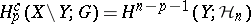is valid for any subset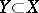(Sitnikov duality). Ifis non-compact one must consider homology with supports closed in all ofrather than homology with compact supports. Ifis compact, reduced homology must be used for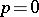.

Non-trivial examples of homology manifolds include "factors" of ordinary manifolds such as Euclidean spaces: If for a topological spacethere exists ansuch that the Cartesian productis a homology manifold, thenandare also homology manifolds. There are examples of homology manifolds that are not locally Euclidean at any one of their points. Homology manifolds play an important role in certain problems of transformation groups (cf. Transformation group), where they appear as orbit spaces or as sets of fixed points.

There exists a cohomological variant of the definition of generalized manifolds. Any cohomology manifold over a principal ideal ring is a homology manifold over, and ifis at most countable, then the converse proposition is true as well.

How to Cite This Entry:
Homology manifold. Encyclopedia of Mathematics. URL: http://encyclopediaofmath.org/index.php?title=Homology_manifold&oldid=14967
This article was adapted from an original article by E.G. Sklyarenko (originator), which appeared in Encyclopedia of Mathematics - ISBN 1402006098. See original article